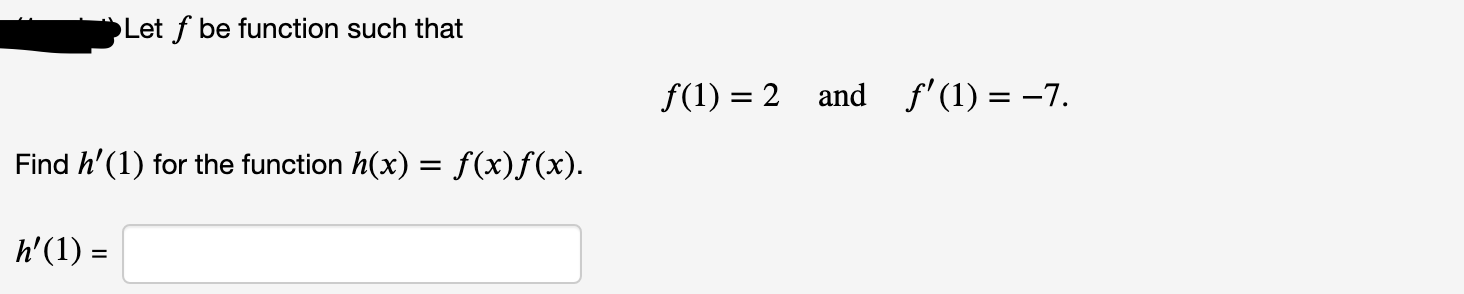# Let f be function such that f(1) = 2 and f'(1) = -7. Find h'(1) for the function h(x) = f(x)f(x). h'(1) = %3D

Questionhelp_outlineImage TranscriptioncloseLet f be function such that f(1) = 2 and f'(1) = -7. Find h'(1) for the function h(x) = f(x)f(x). h'(1) = %3D fullscreen

### Want to see this answer and more?

Experts are waiting 24/7 to provide step-by-step solutions in as fast as 30 minutes!*

*Response times may vary by subject and question complexity. Median response time is 34 minutes for paid subscribers and may be longer for promotional offers.
Tagged in
MathCalculus

### Derivative